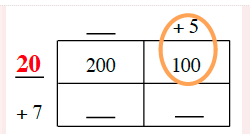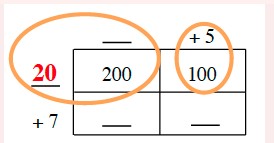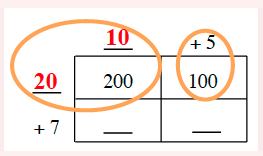### Home > MC1 > Chapter 3 > Lesson 3.4.3 > Problem3-121

3-121.

Copy and complete the generic rectangle below. What multiplication problem does it represent and what is the product?

 _____ $+5$ _____ $200$ $100$ $+7$ _____ _____

2 by 2 generic rectangle edges labeled as follows: Top edge left, blank, top edge right, + 5. Left edge top, blank, left edge bottom, + 7. Interior area labeled as follows: top left, 200, top right, 200, bottom left, blank, bottom right, blank.

You know that $5(\text{something}) = 100$. What is that something?

Added to the rectangle: left edge top is 20. A circle around +5 and 100.

You know that $20(\text{something}) = 200$. What is that something?

Circle added around left edge top, 20, top edge left ,blank, and top left interior, 200.

Complete the rest of the square using multiplication. Added to rectangle & inside second circle: top edge left is 10.